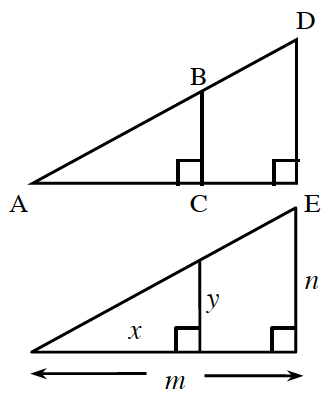### Home > A2C > Chapter 1 > Lesson 1.1.3 > Problem1-40

1-40.

Consider triangles $ABC$ and $ADE$ at right. Give a convincing argument why $∆ABC\sim∆ADE$. Then use what you know about similar triangles to complete each of the following ratios for the triangles.

1. $\frac { y } { x } = \frac { ? } { ? }$

Notice that $y$ and $x$ are the longer and shorter legs of $ΔABC$.

Remember that $ΔABC$ and $ΔADE$ are similar, which means their sides are proportional.

$\frac{y}{x}=\frac{n}{m}$

1. $\frac { n } { y } = \frac { ? } { ? }$

Notice that both $n\ \text{and}\ y$ are the short legs in both triangles